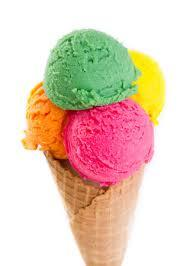# Strawberry 4312

Half of the class likes vanilla ice cream, half of the rest like strawberry, and the other six like hazelnut. How many children were there in the class?

n =  24

### Step-by-step explanation:

n/2+1/2•(n-n/2)+6 = n

0.5n = 12

n = 24

Our simple equation calculator calculates it.Did you find an error or inaccuracy? Feel free to write us. Thank you!

Tips for related online calculators
Do you have a linear equation or system of equations and looking for its solution? Or do you have a quadratic equation?